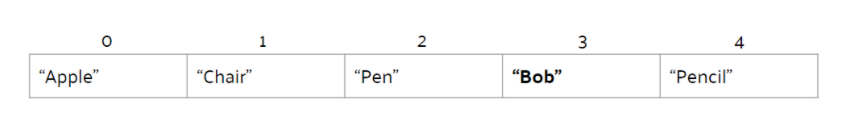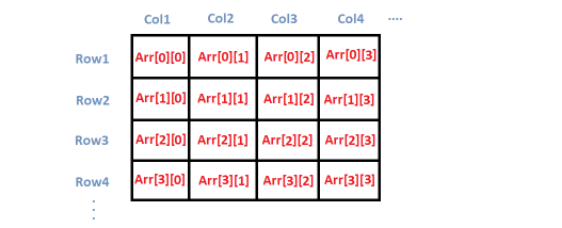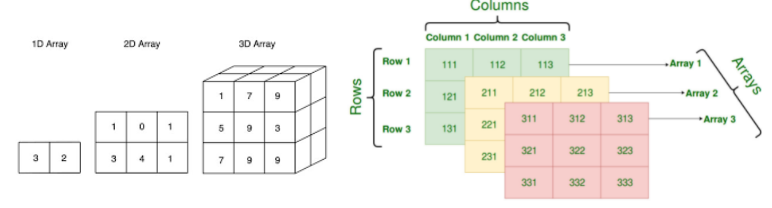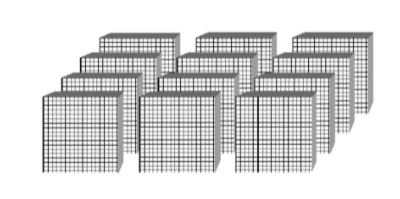# Multi-Dimensional Arrays

### Array Review

If you’ve been tuning in to the Competitive Branch’s lessons, you probably have had some sort of introduction to arrays. We’ll give another quick rundown of arrays and then hop right into multi-dimensional arrays.

##### Concept of Arrays

Arrays are an arrangement of items of a certain data type. These can range from integers, floating point numbers, strings, and even objects. You can think of this as a sort of line of items, and you can items at any position. Arrays are zero-indexed, meaning that the elements are indexed `0, 1, 2...`. In our example below, the fourth element `"Bob"` with index 3 is bolded.Here is the declaration of an array in Java:

``````//data_type represents the data type of the array
//var_name is the variable name
//array_size is the size of the array
data_type[] var_name = new data_type[array_size];
int[] array = new int; //integer array of size 30, from indices 0-29
``````
##### Multidimensional Arrays

To explain the concept of multidimensional arrays, let’s start with 2D arrays. This is similar to a spreadsheet, or a table of items. By definition, a 2D array is an “array of arrays”. This means that it is an array with the data type array. The example below demonstrates how 2D arrays work.Here is the declaration of a 2D array in Java:

``````data_type[][] var_name = new data_type[array_size1][array_size2];
String[][] names = new String; //2d string array with 4 * 5 = 20 elements
``````

A 3D array is an array of 2D arrays. You can visualize this as a cube, or a collection of indexed 2D arrays. Below shows a comparison between the three and shows more deeply what a 3D array would look like.Here is the declaration of a 3D array in Java:

``````data_type[][][] var_name = new data_type[array_size1][array_size2][array_size3];
float[][][] values = new float; //3d float array with 2 * 5 * 20 = 200 elements
``````

A 4D array would be a collection of 3D arrays, or an array of 3D arrays. Trying to visualize this in four dimensions is pretty hard, so we can instead think of it as a bunch of 3D blocks with numbers attached to each of them.Here is the declaration of a 4D array in Java:

``````data_type[][][][] var_name = new data_type[array_size1][array_size2][array_size3][array_size4];
Student[][][][] students = new Student; //4D Student class array with 1 * 2 * 3 * 4 = 24 elements
``````